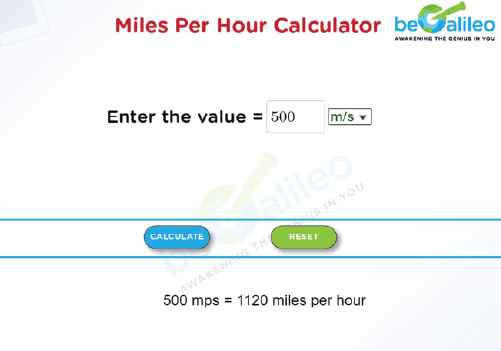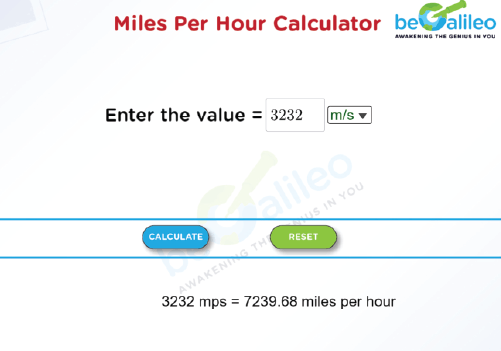>### Master Math with 1:1 Online Classes. Learn from Experts!# Miles Per Hour Calculator

A Miles per Hour Calculator is an online tool that converts the speed from kilometers per hour or meters per second to miles per hour. Miles per hour (mph) is the number of miles traveled per hour.

The calculator takes an input value and converts it into mph based on a conversion factor.

• The conversion factor to convert kilometers per hour to miles per hour is 1.61, which means that one mile per hour is equal to 1.61 kilometers per hour.
• The conversion factor to convert meters per second to miles per hour is 2.24, which means that 1 meter per second is equal to 2.24 miles per hour.
Meters per second < Miles per hour < Kilometres per hour

Miles per hour calculator is a useful tool in many fields, including transportation, sports, and recreation.

## How to use the Miles Per Hour Calculator?

1. Entering the value :
The speed value should be entered in the text box and its unit km/h or m/s must be selected from the drop-down box.
2. Calculation :
Click on "CALCULATE" and the speed value will be converted into miles per hour and displayed on the screen.
3. Stepping into the next :
Click "RESET".
The previous calculations will be erased so that the new speed value can be entered.

## How to convert the speed of other units into Miles Per Hour?

1. Conversion of Kilometers per hour to Miles per hour.
Miles per hour = \frac{\text{km per hour}}{1.61}
This formula is used for the conversion of speed from km/hr to miles per hour.
2. Conversion of Meters per second to Miles per hour.
Miles per hour = Meters per second \times 2.24
This formula is used for the conversion of speed from m/s to miles per hour.

## Solved Examples :

### Q1. Convert 500 meters per second into miles per hour.

Solution :
Given, Speed = 500 meters per second
Using the formula, Miles per hour=\text{Meters per second} \times2.24
= 500 \times 2.24 = 1120 \text{ miles per hour}### Q2. Convert 3232 kilometers per hour into miles per hour.

Solution :
Given, Speed = 3232 kilometers per hour
Using the formula, Miles per hour=\frac{km\ per\ hour}{1.61}
=\frac{3232}{1.61}=2007.45 \text{ miles per hour}